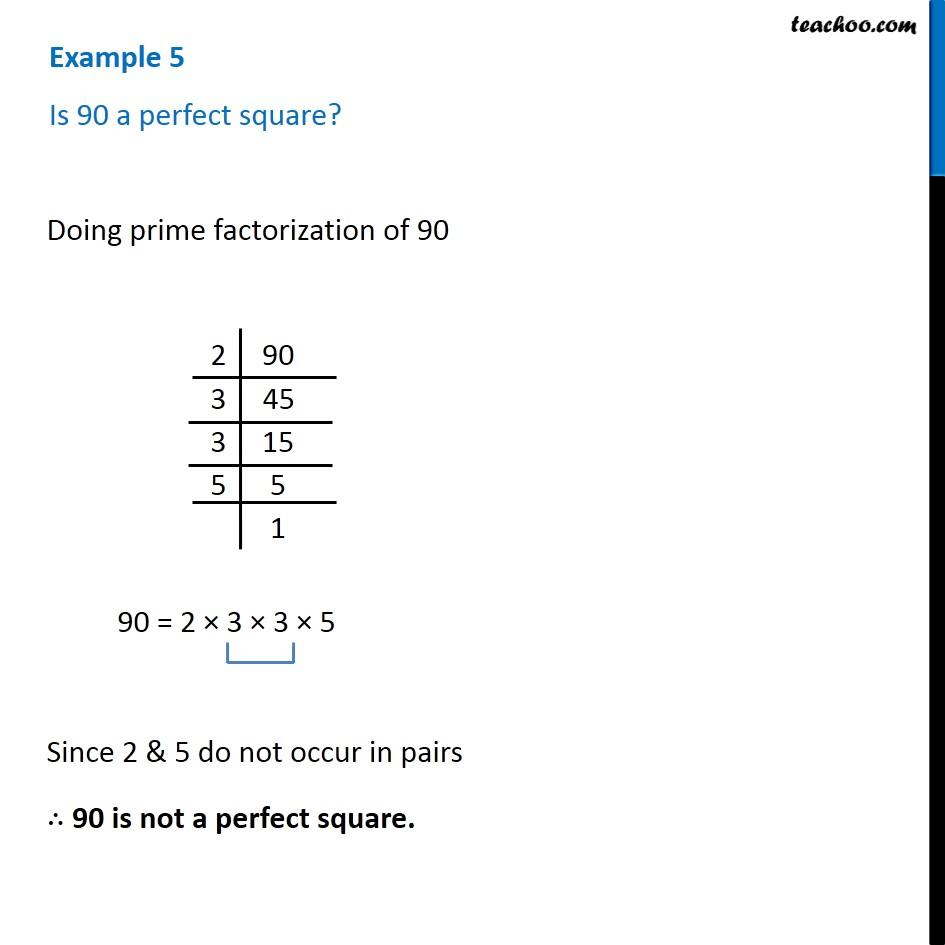1. Chapter 6 Class 8 Squares and Square Roots
2. Serial order wise
3. Examples

Transcript

Example 5 (Chapter 6 Class 8 Square and Square Roots NCERT Book) Is 90 a perfect square? Doing prime factorization of 90 2 | 90 3 | 45 3 | 15 5 | 5 | 1 Thus, 90 = 2 × 3 × 3 × 5 Since 2 & 5 do not occur in pairs ∴ 90 is not a perfect square.

Examples